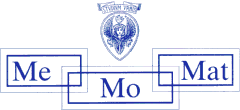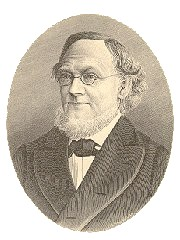# Grassmann Spaces

We exhibit the geometry and embeddings of the Grassmann space G(n,d) formed by the d-dimensional subspaces of an n-dimensional projective space over an arbitrary (skew) field F and related topics, like products of Grassmann spaces, Schubert spaces, or flag spaces.## Generalised Schubert Varieties

Italian Head of Project: Alessandro Bichara
Dipartimento di Metodi e Modelli Matematici, Università di Roma "La Sapienza"

Austrian Head of Project: Hans Havlicek

Fourth Protocol of Scientific and Technological Cooperation between Italy and Austria (project no. 10).Hermann Graßmann

## Affine Spaces from Grassmann Spaces

If the ground field F is commutative then the Grassmann space G(3,1) admits a well known point model, namely the Klein quadric lying in a 5-dimensional projective space. Via a stereographic projection a proper subset of the Klein quadric can be mapped bijectively onto a 4-dimensional affine space. So all lines that are skew to a fixed line become the points of a 4-dimensional affine space.

If the ground field F is a proper skew field then there is no point model like the Klein quadric. However, it is still possible to endow the set of all lines that are skew to a fixed line with the structure of an affine space. Thus the construction from above can be carried out irrespective of the existence of the Klein quadric. From a local point of view this affine space may serve as a substitute for the Klein quadric.

Similar constructions are possible for higher dimensions.

## Publications

• H. Havlicek: Zur Theorie linearer Abbildungen I, J. Geometry 16 (1981), 152-167.
• H. Havlicek: Zur Theorie linearer Abbildungen II, J. Geometry 16 (1981), 168-180.
• H. Havlicek: Liniengeometrische Modelle affiner 4-Räume, In: N.K. Stephanidis (ed.): Proceedings of the Congress of Geometry - Thessaloniki 1987, 47-53.
• H. Havlicek: On sets of lines corresponding to affine spaces In: A. Barlotti et al. (eds.): Combinatorics '88, Proceedings of the International Conference on Incidence Geometries and Combinatorial Structures (Ravello, May 1988), vol. 1, Research and Lecture Notes in Mathematics, Mediterranean Press 1991, 449-457.
Preprint (PDF)
• H. Havlicek: Baer subspaces within Segre manifolds, Results Math. 23 (1993), 322-329.
Preprint (PDF)
• H. Havlicek: On isomorphisms of Grassmann spaces, Mitt. Math. Ges. Hamburg 14 (1995), 117-120.
Preprint (PDF)
• A. Blunck, H. Havlicek: Affine spaces within projective spaces, Resultate Math. 36 (1999), 237-251.
Preprint (PDF)
• H. Havlicek, K. List, and C. Zanella: On automorphisms of flag spaces, Linear Multilinear Algebra 50 (2002), 241-251.
Preprint (PDF)
• A. Bichara, H. Havlicek, and C. Zanella: On linear morphisms of product spaces, Discrete Math. 267 (2003), 35-43.
Preprint (PDF)
• H. Havlicek, C. Zanella: On embedded products of Grassmannians, Discrete Math. 267 (2003), 153-158.
Preprint (PDF)
• A. Blunck and H. Havlicek: On bijections that preserve complementarity of subspaces, Discrete Math. 301 (2005), 46-56.
Preprint (PDF)
• H. Havlicek, V. Pambuccian: On the axiomatics of projective and affine geometry in terms of line intersection, Resultate Math. 45 (2004), 35-44.
Preprint (PDF)
• H. Havlicek and M. Pankov: Transformations on the product of Grassmann spaces, Demonstratio Math. 38 (2005), 675-688.
Preprint (PDF)

Andrea Blunck
Hans Havlicek
Klaus List